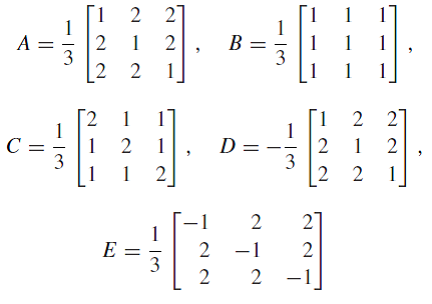Ask question

# True or FalseIf A and B are nxxn lower triangular matrices, then AB is also lower triangular# True or FalseIf A and B are nxxn lower triangular matrices, then AB is also lower triangular

Question
Matricesasked 2021-03-05

True or False If A and B are $$n \times n$$ lower triangular matrices, then AB is also lower triangular

## Answers (1)2021-03-06

Consider 2 x 2 lower triangular matrix

$$\displaystyle{A}={\left[\begin{matrix}{a}&{0}\\{b}&{c}\end{matrix}\right]}{\quad\text{and}\quad}{B}={\left[\begin{matrix}{d}&{0}\\{e}&{f}\end{matrix}\right]}$$

Now taking multiplication of A and B we get,

$$\displaystyle{A}{B}={\left[\begin{matrix}{a}&{0}\\{b}&{c}\end{matrix}\right]}{\left[\begin{matrix}{d}&{0}\\{e}&{f}\end{matrix}\right]}$$

$$\displaystyle{A}{B}={\left[\begin{matrix}{a}{d}&{0}\\{b}{d}+{c}{e}&{c}{f}\end{matrix}\right]}$$

Therefore, AB is also an upper triangular matrix, N

Hence, if A and B are n x n lower triangular matrices, then AB is also lower triangular. Therefore, given statement is true.

### Relevant Questionsasked 2020-11-30

Let M be the vector space of $$2 \times 2$$ real-valued matrices.
$$M=\begin{bmatrix}a & b \\c & d \end{bmatrix}$$
and define $$M^{\#}=\begin{bmatrix}d & b \\c & a \end{bmatrix}$$ Characterize the matrices M such that $$M^{\#}=M^{-1}$$asked 2020-11-30
True or False
If A and B are $$n \times n$$ lower triangular matrices, then AB is also lower triangularasked 2021-03-26

One of the five given matrices represents an orthogonal projection onto a line and another represents a reflection about line. Identify both and briefly justify your choice.asked 2020-10-25

Solve for X in the equation, given
$$3X + 2A = B$$
$$A=\begin{bmatrix}-4 & 0 \\1 & -5\\-3&2 \end{bmatrix} \text{ and } B=\begin{bmatrix}1 & 2 \\ -2 & 1 \\ 4&4 \end{bmatrix}$$asked 2021-02-24

Let $$u=\begin{bmatrix}2 \\ 5 \\ -1 \end{bmatrix} , v=\begin{bmatrix}4 \\ 1 \\ 3 \end{bmatrix} \text{ and } w=\begin{bmatrix}-4 \\ 17 \\ -13 \end{bmatrix}$$ It can be shown that $$4u-3v-w=0$$. Use this fact (and no row operations) to find a solution to the system $$4u-3v-w=0$$ , where
$$A=\begin{bmatrix}2 & -4 \\5 & 17\\-1&-13 \end{bmatrix} , x=\begin{bmatrix}x_1 \\ x_2 \end{bmatrix} , b=\begin{bmatrix}4 \\ 1 \\ 3 \end{bmatrix}$$asked 2021-01-04
In the following question there are statements which are TRUE and statements which are FALSE.
Choose all the statements which are FALSE.
1. If the number of equations in a linear system exceeds the number of unknowns, then the system must be inconsistent - thus no solution.
2. If B has a column with zeros, then AB will also have a column with zeros, if this product is defined.
3. If AB + BA is defined, then A and B are square matrices of the same size/dimension/order.
4. Suppose A is an n x n matrix and assume A^2 = O, where O is the zero matrix. Then A = O.
5. If A and B are n x n matrices such that AB = I, then BA = I, where I is the identity matrix.asked 2021-03-02
Zero Divisors If a and b are real or complex numbers such thal ab = O. then either a = 0 or b = 0. Does this property hold for matrices? That is, if A and Bare n x n matrices such that AB = 0. is il true lhat we must have A = 0 or B = 0? Prove lhe resull or find a counterexample.asked 2021-02-19
Determine whether each of the following statements is true or false, and explain why.If A and B are square matrices of the same size, then AB = BAasked 2020-12-29
If A and B are $$n \times n$$ diagonalizable matrices , then A+B is also diagonalizable.
True or False?asked 2021-05-18
The student engineer of a campus radio station wishes to verify the effectivencess of the lightning rod on the antenna mast. The unknown resistance $$\displaystyle{R}_{{x}}$$ is between points C and E. Point E is a "true ground", but is inaccessible for direct measurement because the stratum in which it is located is several meters below Earth's surface. Two identical rods are driven into the ground at A and B, introducing an unknown resistance $$\displaystyle{R}_{{y}}$$. The procedure for finding the unknown resistance $$\displaystyle{R}_{{x}}$$ is as follows. Measure resistance $$\displaystyle{R}_{{1}}$$ between points A and B. Then connect A and B with a heavy conducting wire and measure resistance $$\displaystyle{R}_{{2}}$$ between points A and C.Derive a formula for $$\displaystyle{R}_{{x}}$$ in terms of the observable resistances $$\displaystyle{R}_{{1}}$$ and $$\displaystyle{R}_{{2}}$$. A satisfactory ground resistance would be $$\displaystyle{R}_{{x}}{<}{2.0}$$ Ohms. Is the grounding of the station adequate if measurments give $$\displaystyle{R}_{{1}}={13}{O}{h}{m}{s}$$ and R_2=6.0 Ohms?
...